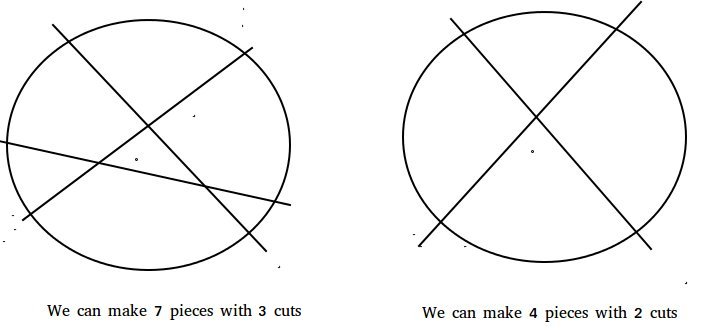# Pizza cut problem (Or Circle Division by Lines)

Given number of cuts, find the maximum number of possible pieces.

Examples:

```Input  : 2
Output : 4

Input  : 3
Output : 7```

## Recommended: Please try your approach on {IDE} first, before moving on to the solution.

This problem is nothing but The Lazy Caterer’s Problem and has below formula.

Maximum number of pieces = 1 + n*(n+1)/2

Refer this for proof.

## C++

 `// C++ program to find maximum no of pieces ` `// by given number of cuts ` `#include ` `using` `namespace` `std; ` ` `  `// Function for finding maximum pieces ` `// with n cuts. ` `int` `findMaximumPieces(``int` `n) ` `{ ` `   ``return` `1 + n*(n+1)/2;     ` `} ` ` `  `// Driver code ` `int` `main() ` `{ ` `   ``cout << findMaximumPieces(3); ` `   ``return` `0; ` `} `

## Java

 `// Java program to find maximum no of  ` `// pieces by given number of cuts ` `class` `GFG { ` `     `  `    ``// Function for finding maximum pieces ` `    ``// with n cuts. ` `    ``static` `int` `findMaximumPieces(``int` `n)  ` `    ``{ ` `        ``return` `1` `+ n * (n + ``1``) / ``2``;  ` `    ``} ` `     `  `    ``// Driver Program to test above function ` `    ``public` `static` `void` `main(String arg[])  ` `    ``{ ` `         `  `        ``System.out.print(findMaximumPieces(``3``)); ` `    ``} ` `} ` ` `  `// This code is contributed by Anant Agarwal. `

## Python3

 `# Python3 program to find maximum ` `# no. of pieces by given ` `# number of cuts ` ` `  `# Function for finding maximum ` `# pieces with n cuts. ` `def` `findMaximumPieces(n): ` `    ``return` `int``(``1` `+` `n ``*` `(n ``+` `1``) ``/` `2``) ` ` `  `# Driver code ` `print``(findMaximumPieces(``3``)) ` ` `  `# This code is contributed 29AjayKumar `

## C#

 `// C# program to find maximum no of  ` `// pieces by given number of cuts ` `using` `System; ` ` `  `class` `GFG { ` `      `  `    ``// Function for finding maximum pieces ` `    ``// with n cuts. ` `    ``static` `int` `findMaximumPieces(``int` `n)  ` `    ``{ ` `        ``return` `1 + n * (n + 1) / 2;  ` `    ``} ` `      `  `    ``// Driver Program to test above function ` `    ``public` `static` `void` `Main()  ` `    ``{ ` `          `  `        ``Console.Write(findMaximumPieces(3)); ` `    ``} ` `} ` `  `  `// This code is contributed by nitin mittal. `

## PHP

 ` `

Output:

```7
```

This article is contributed by DANISH_RAZA. If you like GeeksforGeeks and would like to contribute, you can also write an article using contribute.geeksforgeeks.org or mail your article to contribute@geeksforgeeks.org. See your article appearing on the GeeksforGeeks main page and help other Geeks.

Attention reader! Don’t stop learning now. Get hold of all the important DSA concepts with the DSA Self Paced Course at a student-friendly price and become industry ready.

My Personal Notes arrow_drop_up

Improved By : nitin mittal, 29AjayKumar

Article Tags :
Practice Tags :

3

Please write to us at contribute@geeksforgeeks.org to report any issue with the above content.